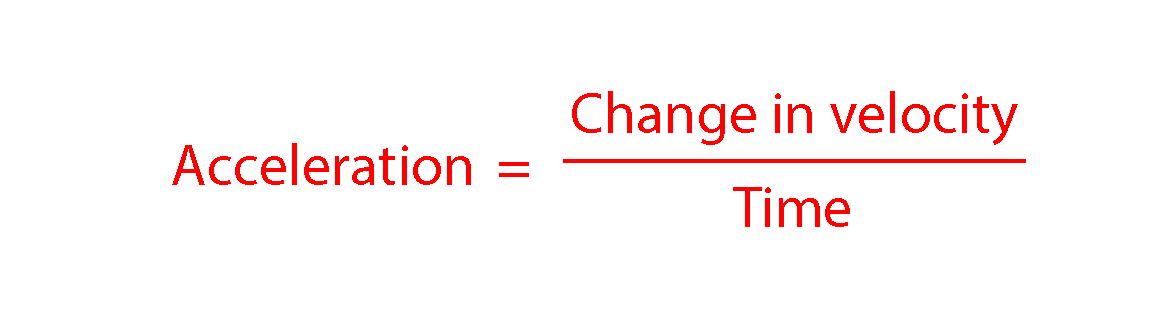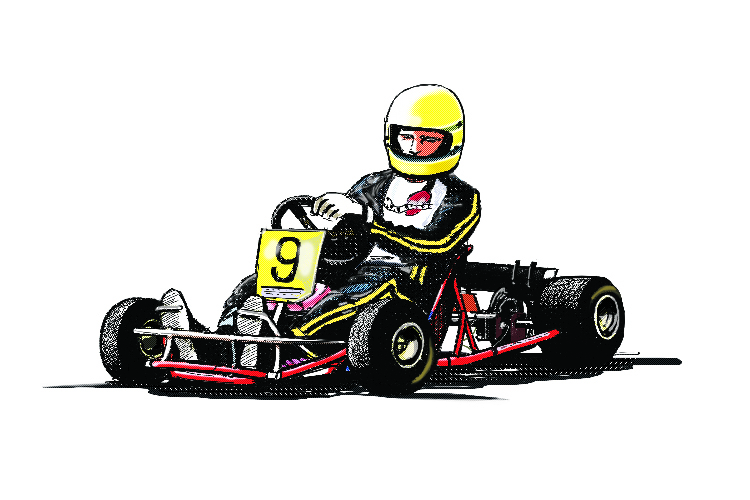# Acceleration

Acceleration is a change in velocity. However it can sometimes be easier to think of it as a change in speed. How much an object accelerates depends on how quickly its velocity/speed changes.

The units for acceleration are usually metres per second per second (m/s/s) or metres per second squared (m/s2).

The formula used to calculate acceleration is:Ac\c\e\l\e\r\ation=((v_2-v_1))/t

You may also see this written as

Ac\c\e\l\e\r\ation=(Deltav)/tAccelerator pedal to the floor changes speed to stay on time.

Example 1

A cyclist increases her speed from 5m//s to 19m//s in 7s. What is her acceleration?

Ac\c\e\l\e\r\ation=(chan\g\e\ \ i\n\ \ velocity)/(time)

Ac\c\e\l\e\r\ation=((19-5))/7=14/7=2\ m//s^2

Example 2

An oil tanker decelerates at an average rate of 0.4m//s^2. How long to the nearest minute would it take to come to a complete stop if it was initially travelling at 12m//s?

Ac\c\e\l\e\r\ation=(chan\g\e\ \ i\n\ \ velocity)/(time)

-0.04=((0-12))/(time)

time=((0-12))/-0.04=(-12)/-0.04=300\ \ se\c\o\nds

300/60=5\ m\i\n\u\tes

Example 3

A goalkeeper takes a goal kick and the ball travels away from goal. When it reaches an opposing striker at a velocity of 12.4m//s the ball is kicked back towards goal by the striker. This results in the ball accelerating at 72m//s^2 towards the goal for the 0.45 seconds during which the striker's foot is in contact with the ball. At what velocity does the ball leave the striker’s foot?

Ac\c\e\l\e\r\ation=(chan\g\e\ \ i\n\ \ velocity)/(time)

Multiply both sides by time to make change in velocity the subject,

Ac\c\e\l\e\r\ationtimestime=(chan\g\e\ \ i\n\ \ velocitytimescancel(time))/(cancel(time))

chan\g\e\ \ i\n\ \ velocity=Ac\c\e\l\e\r\ationtimestime

However there is a trick in this question! The ball is initially traveling away from goal and then accelerates towards goal, this means the acceleration is in the opposite direction and therefore is in a negative direction!

chan\g\e\ \ i\n\ \ velocity=-72times0.45=-32.4\ m//s

chan\g\e\ \ i\n\ \ velocity=v_2-v_1

v_2-12.4=-32.4\ m//s

v_2=-32.4+12.4=-20\ m//s

NOTE:

The velocity is negative as the direction the ball travels after the striker kicks it is opposite to the direction it was travelling when the goalkeeper kicked it.